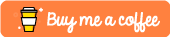Variables in Python

Let’s first have a look at variables. A variable is essentially a place where we can store the value of something for processing later on. Imagine you wanted to write a program that doubled a number for us, not the most exciting of programs I know but it is a good example.

We would first want to define a variable to store our number, double it and then print it out.

Open up visual studio code and create a new python file called double.py. In this double.py file you will want to add the following code:

my_number = 2
print(my_number)

my_number = my_number * 2
print(my_number)

We start off by defining a new variable called my_number and setting this new variable to equal 2. After this we then print out the value of our variable using the print function and passing in what we wish to print, in this case we wish to print our my_number variable.

When you run this you should see the number 2 being printed out in the console.

>>> my_number = 2
>>> print(my_number)
2

After this we then re-assign our my_number variable to equal the current value of my_number times 2. This doubles our starting number. When we now run this program you should see the number 4 being printed out below our 2 value.

>>> my_number = 2
>>> print(my_number)
2
>>> my_number = my_number * 2
>>> print(my_number)
4

Exercise - Try changing the my_number variable to a series of different numbers to test this out.

Now this example may work with numeric values, but what happens if we want to define a variable that stores something like a name? We can do that to simply by choosing a variable name such as first_name and then setting this to equal the string of our choice surrounded by quotes like so:

first_name = "Elliot"
print(first_name)

We can then do cool things like printing out custom messages like this:

print("Welcome: " + first_name)

This should then print out Welcome: Elliot to our console when we run it.

Functions in Python

So now that we’ve got a basic understanding of variables in Python, let’s now move on to functions. If you’ve never encountered functions before then you can think of them as blocks of instructions, imagine you had a maths program that could either double or triple a number of your choice. You would want to define two distinct functions that would take in a number and either double or triple it.

In order to define functions in python we can use the def keyword followed by the name of the function we wish to define like so:

def double(my_number):

This is known as a function declaration and it’s below this function declaration that we’ll define what we want our function to do. You should notice that we’ve included (my_number) beside our function, this is how we define what goes into every function. In this case we want it to take in a my_number variable which we will then go on to double within our function.

def double(my_number):
return my_number * 2

Note: we have used the return keyword which returns the value of my_number * 2 whenever this function is called.

If we then wished to use this function then we could do something like this:

def double(my_number):
return my_number * 2

# create a new variable called `my_var` which equals
# the value returned by calling double() on the value 5.
my_var = double(5)
# print our new `my_var`
print(my_var)

Exercise - Try implementing a triple function that takes in a my_number variable and returns triple this value.

Further Examples

In the previous example we defined a pretty small function that only featured one line of code within its body. It’s important to note however that you can define many lines of code within your function and even call other functions from within your own function. There is also no rule that states you must return something from within your function. It could, for example, just perform a series of print statements like so:

def test_function():
print("Hello World")
print("This is a multi-line function")
print("that does not return anything")